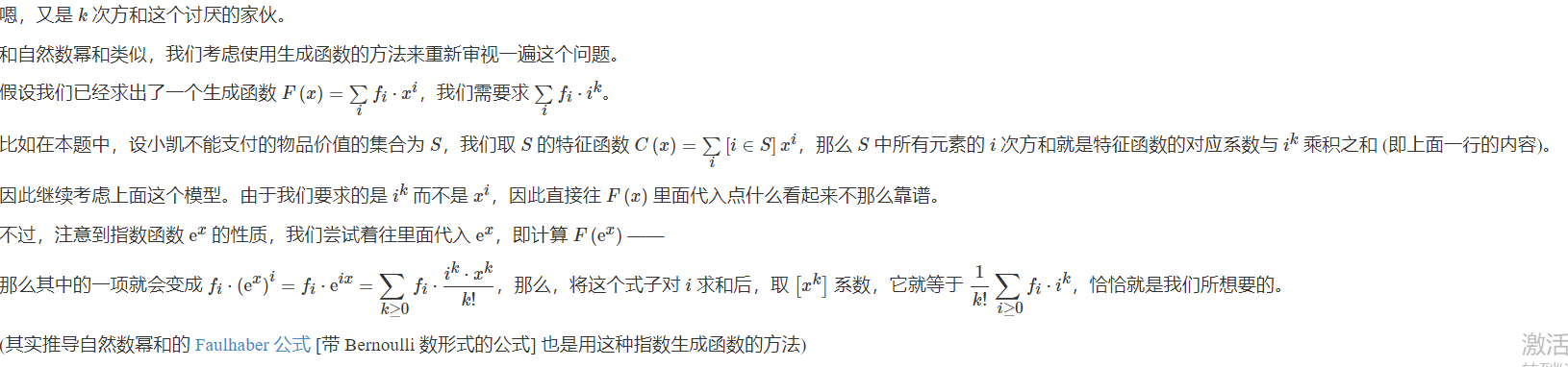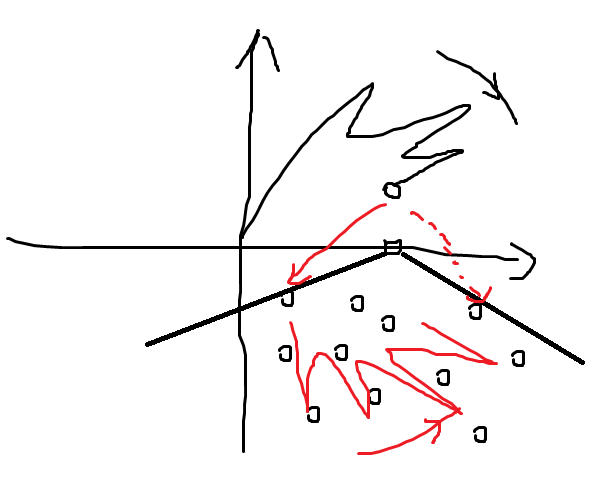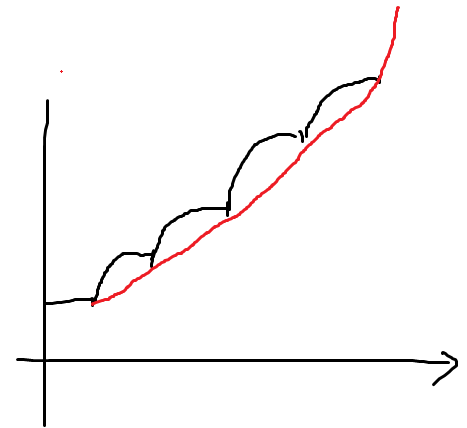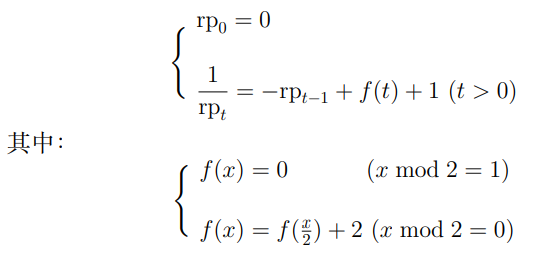# 7029. 2021.3.27CCF NOI Online 能力测试提高组第一题 愤怒的小 N# PE753 Fermat Equation

$$x^3+y^3\equiv 1 \pmod p$$的解的对数为$$p-2+A$$

# 7033. 2021.03.28【2021省赛模拟】超命运树 (destsurr)

①只有一个儿子选

1、超集另一边不选，所以i可选可不选，且超集的选择相同

2、超集另一边选（只放一个所以乘size），所以i必选否则Len不对

②两个儿子选

i必不能选否则f不对，超集可选可不选

597上次出的题的超集

# PE670 Colouring a Strip/PE671 Colouring a Loop

670好做，直接矩乘加速f[i,0~3,0~3]，表示匹配了多少位

671先Burnside（其实n是质数所以不用），然后变成环了所以加上两维变成f[i,0~3,0~3,0~2,0~2]，表示颜色是否与初始的两种相同，直接矩乘+亿点点细节

# PE742 Minimum area of a convex grid polygon

dp1/4的轮廓线，把<=50的(x,y)=1的向量xy找出来，高度最多到3000即可

# PE741 Binary grid colouring

rk95，好耶

Burnside，变成不动、转90、转180、沿边翻转、沿对角线翻转

①沿边翻转

②不动

③转90

④沿对角线翻转

⑤转180

# 6084. 【GDOI2019模拟2019.3.25】礼物

burnside，然后对于每段枚举首+尾长度，中间组合数+容斥，暴力枚举是O(Σσ0)，把组合数合并变为O(Σσ0/K)

# PE317 Firecracker

$$\large 1/2(2v-gt)t=h$$

$$\large 1/2(2v\sin-gs\sec /v)s\sec=h$$

$$\large \sec'=\tan\sec$$，则

$$h'=\large s/2(2v\sec^2-gs\tan\sec^2 /v)=0$$，中间有一步$$\large \tan^2+1=\sec^2$$

ans=正-最小割

# 2021.03.15【2021省赛模拟】C

ll的做法：先找叶子，然后每个点二分出编号最大的叶子，等于按叶子从小到大access，之后对每条链排序，再按链编号从小到大二分儿子

# CF1500D. Tiles for Bathroom

O(n^2dlogn)的做法：确定左上角二分边长，用倍增搞出2^k大小的矩形，在不能*2时把四个矩形合并判断

CF上注意空间

# 5680. 【GDOI2018Day2模拟4.21】绝对伏特加

$$=\large [\frac{x^n}{n!}] (\sum_k S[F,k] x^ke^x)^Le^{(K-L)x}$$

$$=\large [\frac{x^n}{n!}] (\sum_k S[F,k] x^k)^Le^{Kx}$$

# 7004. 2021.03.11【2021省赛模拟】洛希极限（roche）

upd：一开始求往右和往下可以并查集O(nm)，之后的单调队列满足右端点不减（矩形包含），所以可以直接搞

# agc052

A：直接n个0+n个1+0即可，若s[n]为0则显然，否则找到前面的第一个0，中间的1可以在第一个s时匹配掉

B：新建虚点连1，发现操作等于交换两个点到虚点的xor距离，而距离和边权一一对应，所以二者等价

C：当和%p=0显然无解，否则考虑把众数x乘逆元变为1，则有解的充要条件为 1的个数<=(p-1)+Σp-大于1的数

D：先求lis，若长2k则把f<=k的和>k的分成两组，否则为2k+1，由于不能在内部分，所以一定有一个非2k+1里面的被选，设其为x，则有解的充要条件为x在一条>=k+1链里

E：把S变为数组a，满足相邻绝对值=1且ABC对应%3=012，发现只要确定a1后面唯一确定，同时若S->T则反过来操作a与S互相对应，所以只需要考虑所有a1即可

# CF1500C. Matrix Sorting

597的做法：从后往前做，每次把有序的划分集合，与划分顺序无关，维护一下每个元素的最长结尾上升子串即可O(n^2)

# 牛客练习赛77F 小G的排列

dp，设f[i,j]表示放了i段总长j，随便写有n^3

# 6653. 【2020.05.27省选模拟】树

2 3 4 5 6 7 8 9 10

1 1 1 2 2 2 3 3 3

2 3 4 5 6 7 8 9 10

1 2 3 4 5 6 7 7 7

# 6652. 【2020.05.27省选模拟】序列

nm^2做法：考虑每个序列中每个数的移动方向，发现对于任意两种数，其在两个序列的关系为ab,ab，则两个b的移动方向相同；若为ab,ba，则后面的b和a移动方向相反，并查集连边最后构造

# uoj#604. 【UER #9】赶路（鬼知道double怎么过的

# gym101239E. Evolution in Parallel

①祖先关系可以传递，若a不是b的祖先则a的后代也不是b的祖先

②合法方案中，任意三个必存在一对祖先后代关系

# CF1307G. Cow and Exercise

$$min(\sum x_{ij})$$

$$\left\{\begin{matrix} d_j \leq d_i+w_{ij}+x_{ij} \\ d_T-d_S \geq ans\\ x_{ij}\geq0,d无限制 \end{matrix}\right.$$

$$max(\sum f_{ij}w_{ij}+F*ans)$$

$$\left\{\begin{matrix} -f_{ij}\leq 1\\ \sum f_{ix}-\sum f_{xi}=0\ \ \\ \sum f_{ix}-\sum f_{xi}-F=0\ \ (x=S) \\ \sum f_{ix}-\sum f_{xi}+F=0\ \ (x=T) \\ f_{ij}\leq 0,F\geq 0 \end{matrix}\right.$$# 6991. 2021.2.20【2021省赛模拟】仲夏夜跑步

$2f_i=\sum_j \binom{i-1}{j}f_jf_{i-j-1}，把f变为EGF得\\ 2if_i=\sum_j f_jf_{i-j-1}\\ 2F'=F^2+1\\ 2\frac{dF}{dx}=F^2+1\\ 2\frac{dF}{F^2+1}=dx\\ 2arctan(F)=x+C\\ F=tan(\frac{x+C}{2})\\ 由于F(0)=1，所以C=\pi/2\\ F=\frac{cosx}{1-sinx}\\$

$$sinx'=cosx$$$$cosx'=-sinx$$，设Δ然后展开

$$\large sinx=\frac{x^1}{1!}-\frac{x^3}{3!}+\frac{x^5}{5!}...$$

$$\large cosx=\frac{x^0}{0!}-\frac{x^2}{2!}+\frac{x^4}{4!}...$$

$$tan'(x)=\frac{1}{cos^2(x)}$$$$tan'(x)=(\frac{sinx}{cosx})'=\frac{cos^2x+sin^2x}{cos^2x}=\frac{1}{cos^2x}$$

$$arctan'(x)=\frac{1}{x^2+1}$$

$$设y=arctan(x),x=tan(y)$$
$$y'=\frac{dy}{dx}=\frac{1}{\frac{dx}{dy}}=\frac{1}{tan'(y)}=cos^2(y)=\frac{1}{x^2+1}$$

$$x^2=\frac{sin^2}{cos^2}=\frac{1-cos^2}{cos^2},(x^2+1)cos^2=1$$

$$sin2x=2sinxcosx,cos2x=2cos^2x-1,\frac{sinxcosx}{cos^2x}=tanx=\frac{sin2x}{cos2x+1}$$

$$tan(\frac{x+\pi/2}{2})=\frac{sin(x+\pi/2)}{cos(x+\pi/2)+1}=\frac{cosx}{1-sinx}$$

# 6989. 2021.2.20【2021省赛模拟】皇城 pk

$$\large (s+1)!\sum_{i=L}^R \binom{s+k}{s+1}(-1)^{s+k}=[x^{s+1}]-(s+1)!\frac{(-x-1)^{s+R+1}-(-x-1)^{s+L}}{1-(-x)}*\frac{1}{2}$$

# 6988. 2021.2.19【2021省赛模拟】甲鱼漂泊1 2 1 1 3 2 3 1 4 3 5 2 5 3 4 1

# CF1479E. School Clubs

$$\frac{1}{2}(\sum_i \frac{a_i}{n}(g(1)-g(a_i)))+\frac{1}{2}(\sum \sum \frac{a_i}{n}\frac{a_j}{n}(g(a_j+1)-g(a_i)))-\frac{1}{2}\sum \frac{a_i^2}{n^2}(g(a_i+1)-g(a_i))$$

（注意如果换到原来的组里的变化为0，所以最后要减掉）

# arc112F - Die Siedler

$$f(c)=\sum 2^{i-1}(i-1)!c_j$$，则操作后f不变，进一步发现除全0和全2j-1外f和c在<2j下一一对应（当成一个数）

# 6981. 【2021.02.03冬令营模拟】在星河里

m为奇时重心唯一，设M为输入的m，m=M/2，p=M-m-1

$$\large [x^M]\prod \sum_i \binom{i+s-1}{s-1}x^i - \sum_{i>=m+1} \binom{i+s-1}{s-1}x^i$$

$=\large \binom{n+m-1}{n-1} -[x^p]\sum \frac{1}{(1-x)^{n-s}} \sum_{i} \binom{i+m+s}{s-1}x^i$

$=\large \binom{n+m-1}{n-1} -[x^p]\sum \frac{1}{(1-x)^{n-s}} \sum_{i} \binom{i+m+s}{s-1}x^i$

$$=\large \binom{n+m-1}{n-1} - \sum \sum_{i} \binom{i+m+s}{s-1}\binom{p-i+n-s-1}{n-s-1}$$

$$\large =\sum_{i=0}^{s-1} \binom{i+m}{i}\binom{M+n-1-(i+m+1)}{n-1-i}$$

# CF1476F. Lanterns

dp，设f[i]表示处理完前i个能完整覆盖的前缀，转移考虑i往左/右，往右如果f[i-1]覆盖了i就可以往后加，往左一是可以覆盖[i-ai,i-1]，二是可以覆盖某个j满足f[j]+1>=i-a[i]，这样中间的就被覆盖了，所以中间的全部往右，即f[i]=max(k+a[k]),k∈[j+1,i-1]

# 6976. 【2021.02.01冬令营模拟】拉拖机

$$\large\sum_x \sum_y \binom{c}{x}\binom{d}{y}(-1)^{x+y}(q-1)^{xm+yn-xy}q^{cm+dn-cd-xm-yn+xy}$$

posted @ 2021-02-01 16:13  gmh77  阅读(331)  评论(0编辑  收藏  举报